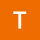Home
IT Knowledge
Inspiration
Languages
EN

# Math - common problems (Cross technology)

5 points
Created by:Trenton-McKinney
318

todo:

TODO:

- create JS math - common problems - related post list + add all math problems to this list

ProblemCrossJS/NodeJavaC#PythonBashPHPC++
Math.sin in degrees
calculate cos in degrees
calculate sin in degrees
Fibonacci sequence
Iterative Fibonacci sequence
calculate root of any degree
get decimal part of float number
round ceil with precision up-to n decimal places example
round floor with precision down-to n decimal places example
round with precision to n decimal places
use Monte Carlo method to calculate pi constant number
use Monte Carlo method to calculate surface area (definite integral)
calculate logarithm with custom base

Note: